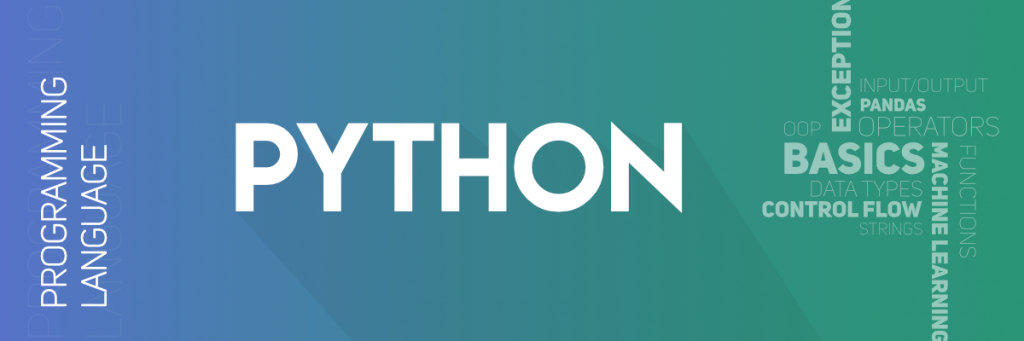Python - Tuples

A tuple is a sequence of immutable Python objects. Tuples are sequences, just like lists. The differences between tuples and lists are, the tuples cannot be changed unlike lists and tuples use parentheses, whereas lists use square brackets.

Creating a tuple is as simple as putting different comma-separated values. Optionally you can put these comma-separated values between parentheses also. For example −

tup1 = ('physics', 'chemistry', 1997, 2000);
tup2 = (1, 2, 3, 4, 5 );
tup3 = "a", "b", "c", "d";

The empty tuple is written as two parentheses containing nothing −

tup1 = ();

To write a tuple containing a single value you have to include a comma, even though there is only one value −

tup1 = (50,);

Like string indices, tuple indices start at 0, and they can be sliced, concatenated, and so on.

Accessing Values in Tuples

To access values in tuple, use the square brackets for slicing along with the index or indices to obtain value available at that index. For example −

#!/usr/bin/python

tup1 = ('physics', 'chemistry', 1997, 2000);
tup2 = (1, 2, 3, 4, 5, 6, 7 );
print "tup1: ", tup1;
print "tup2[1:5]: ", tup2[1:5];

When the above code is executed, it produces the following result −

tup1:  physics
tup2[1:5]:  [2, 3, 4, 5]

Updating Tuples

Tuples are immutable which means you cannot update or change the values of tuple elements. You are able to take portions of existing tuples to create new tuples as the following example demonstrates −

#!/usr/bin/python

tup1 = (12, 34.56);
tup2 = ('abc', 'xyz');

# Following action is not valid for tuples
# tup1 = 100;

# So let's create a new tuple as follows
tup3 = tup1 + tup2;
print tup3;

When the above code is executed, it produces the following result −

(12, 34.56, 'abc', 'xyz')

Delete Tuple Elements

Removing individual tuple elements is not possible. There is, of course, nothing wrong with putting together another tuple with the undesired elements discarded.

To explicitly remove an entire tuple, just use the del statement. For example −

#!/usr/bin/python

tup = ('physics', 'chemistry', 1997, 2000);
print tup;
del tup;
print "After deleting tup : ";
print tup;

This produces the following result. Note an exception raised, this is because after del tup tuple does not exist any more −

('physics', 'chemistry', 1997, 2000)
After deleting tup :
Traceback (most recent call last):
File "test.py", line 9, in <module>
print tup;
NameError: name 'tup' is not defined

Basic Tuples Operations

Tuples respond to the + and * operators much like strings; they mean concatenation and repetition here too, except that the result is a new tuple, not a string.

In fact, tuples respond to all of the general sequence operations we used on strings in the prior chapter −

Python Expression Results Description
len((1, 2, 3)) 3 Length
(1, 2, 3) + (4, 5, 6) (1, 2, 3, 4, 5, 6) Concatenation
('Hi!',) * 4 ('Hi!', 'Hi!', 'Hi!', 'Hi!') Repetition
3 in (1, 2, 3) True Membership
for x in (1, 2, 3): print x, 1 2 3 Iteration

Indexing, Slicing, and Matrixes

Because tuples are sequences, indexing and slicing work the same way for tuples as they do for strings. Assuming following input −

L = ('spam', 'Spam', 'SPAM!')

Python Expression Results Description
L 'SPAM!' Offsets start at zero
L[-2] 'Spam' Negative: count from the right
L[1:] ['Spam', 'SPAM!'] Slicing fetches sections

No Enclosing Delimiters

Any set of multiple objects, comma-separated, written without identifying symbols, i.e., brackets for lists, parentheses for tuples, etc., default to tuples, as indicated in these short examples −

#!/usr/bin/python

print 'abc', -4.24e93, 18+6.6j, 'xyz';
x, y = 1, 2;
print "Value of x , y : ", x,y;

When the above code is executed, it produces the following result −

abc -4.24e+93 (18+6.6j) xyz
Value of x , y : 1 2

Built-in Tuple Functions

Python includes the following tuple functions −

Sr.No. Function with Description
1 cmp(tuple1, tuple2)

Compares elements of both tuples.

2 len(tuple)

Gives the total length of the tuple.

3 max(tuple)

Returns item from the tuple with max value.

4 min(tuple)

Returns item from the tuple with min value.

5 tuple(seq)

Converts a list into tuple.